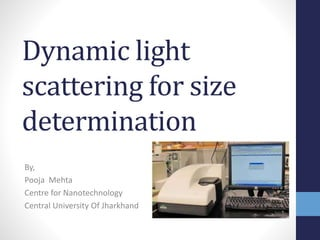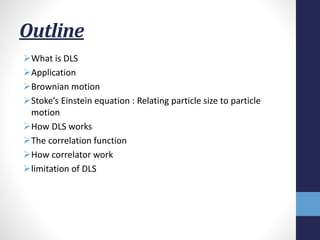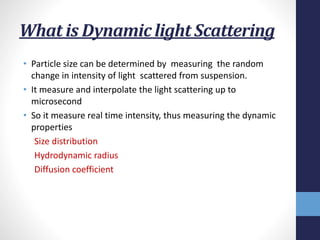Diese Präsentation wurde erfolgreich gemeldet.

# Dynamic Light Scattering

Anzeige
Anzeige
Anzeige
Anzeige
Anzeige
Anzeige
Anzeige
Anzeige
Anzeige
Anzeige
Anzeige×

1 von 20 Anzeige

# Dynamic Light Scattering

Dynamic light scattering (DLS) or Quasi-Elastic Light Scattering (QELS), is a non-invasive, well-established technique for measuring the size and size distribution of molecules and particles typically in the submicron region, and with the latest technology lower than 1nm.
In This slide the working principle and the function of DLS is Explained in brief and precise way.

Dynamic light scattering (DLS) or Quasi-Elastic Light Scattering (QELS), is a non-invasive, well-established technique for measuring the size and size distribution of molecules and particles typically in the submicron region, and with the latest technology lower than 1nm.
In This slide the working principle and the function of DLS is Explained in brief and precise way.

Anzeige
Anzeige

### Dynamic Light Scattering

1. 1. Dynamic light scattering for size determination By, Pooja Mehta Centre for Nanotechnology Central University Of Jharkhand
2. 2. Outline What is DLS Application Brownian motion Stoke’s Einstein equation : Relating particle size to particle motion How DLS works The correlation function How correlator work limitation of DLS
3. 3. What is Dynamic light Scattering • Particle size can be determined by measuring the random change in intensity of light scattered from suspension. • It measure and interpolate the light scattering up to microsecond • So it measure real time intensity, thus measuring the dynamic properties Size distribution Hydrodynamic radius Diffusion coefficient
4. 4. Application Of DLS • We measure Hydrodynamic Size of nanoparticle ,protein and biomaterial • We can also study stability of nanoparticles as function of time • Good for detecting the aggregation of the particles Other Then these • Required small volume of sample • Complete recovery of sample after measurement • Sample preparation is not required for the measurement
5. 5. Brownian motion • Brownian motion is the fundamental of this instrument • Brownian motion of the particle is random motion due to the bombardment by the solvent molecule surround them. • Brownian motion of the particles are related to size. • It describes the way in which very small particles move in fluid suspension
6. 6. Stoke’s Einstein Equation: Relating particle size to particle motion • This random motion is modeled by the Stokes-Einstein equation. d= 𝒌𝑻 𝟑Пŋ 𝑻 𝑫 • d is the hydrodynamic radius of the particle ,the diameter of the sphere that has same diffusion coefficient as the particle. • Temperature of the measurement must be stable ,as the viscosity of the liquid.
7. 7. Hydrodynamic radius • “The size of a hypothetical hard sphere that diffuses in the same fashion as that of the particle being measured”
8. 8. How DLS Works • In DLS we measured the speed at which the particles are diffusing due to Brownian motion. • Speed of diffusion is measured by measuring the rate at which the intensity of the scattered light fluctuates. • Small particles causes the intensity to more fluctuate than larger. • It measure the diffusion coefficient by using correlation function.
9. 9. How these fluctuation in scattered light arises ? Speckle Pattern for a sample containing Stationary Particle For the particle in Brownian motion a speckle pattern is observed where the position of each speckle is seen to be in constant motion Because the phase addition from moving particle is constantly evolving and forming new pattern.
10. 10. Experimental setup of the DLS LASER Attenuator Sample cell Detector Detector 173° 90° Digital signal Processer, Correlator Software for data analyzerScattered light
11. 11. DLS signal Obtained optical signal shows random change due to random change in the position of the particle. The “ noise “ is actually the particle motion and will be used to measure the particle size.
12. 12. Correlation function • A correlation function is statistical correlation between random variables at two different points in space or time, usually as a function of the spatial or temporal distance between the points. • Within the correlation curve all of the information regarding the diffusion of particles within the sample being measured. • Correlator construct correlation function G(τ), of the scattered intensity G(τ)=<I(t).I(t+τ) ,τ is delay time
13. 13. Cont.  If the intensity at time t is compared with the intensity at time t+δt, there will be a strong correlation between two signal.  Correlation of a signal arriving from random source will decrease with time.  If the particle will large the signal will changes slowly and correlation will sustain for long time.
14. 14. Correlogram Typical intensity fluctuations for large and small particles and Corresponding Correlogram
15. 15. How the correlator works • It is a signal comparator. It is design to measure the similarity between two signals or one signals with itself with varying time. • The correlogram give many information the time at which the correlation starts significantly decay is an indication of mean size of the sample • The steeper the curve the more mono disperse the sample is. More extended the decay becomes the greater the polydispesity.
16. 16. Correlation function for monodispresed Particle For monodispersed particle in Brownian motion , the correlation [G] function is an exponential decaying function of decay time τ G(τ) =A[1+Bexp(-2Г τ)] Delay constant (Г) is related to the diffusion coefficient of the particle ,D Г=𝑫𝒒 𝟐 q is the scattering vector which is given by and q= 𝟒П𝒏𝒔𝒊𝒏(Ɵ/𝟐) 𝝀
17. 17. The correlation function for polydisperse particle • G(τ) =A[1+Bexp(2(-Г’ τ+ẚ/2+----)] Г’ is delay constant Г’=𝑫′𝒒 𝟐 D’ is the diffusion coefficent of the And by Polydispersity Index = ẚ 𝟐 Г’ • measure the distribution of size in our sample. • for monodisperse it is about 0.01-0.05 but the value greater than 0.7 indicate that sample has a very broad size distribution.
18. 18. Limitation of DLS • We measure the hydrodynamic radius of the particle, not able to measure the actual size of the particle • The particles having size greater than 1000nm are not measured by this method • Size of Solid particles are not measured by DLS
19. 19. Reference • DLS technical note www.malvern.co.uk • http://www.wyatt.eu/ • Dynamic Light Scattering for Nanoparticle Size Analysis - HORIBA
20. 20. Thank you for your attention